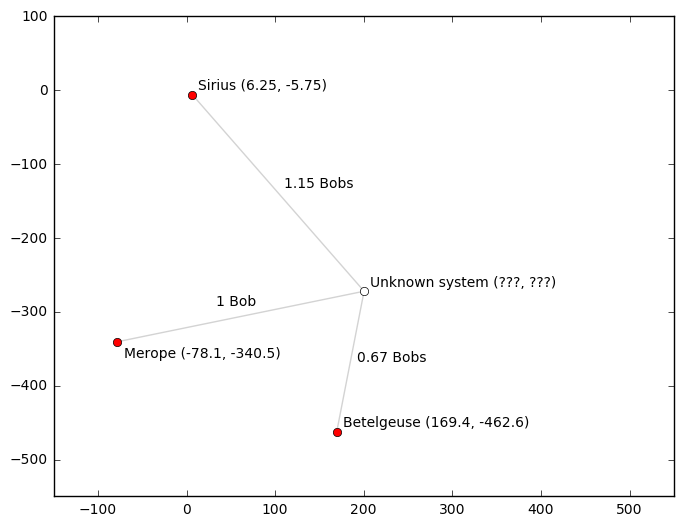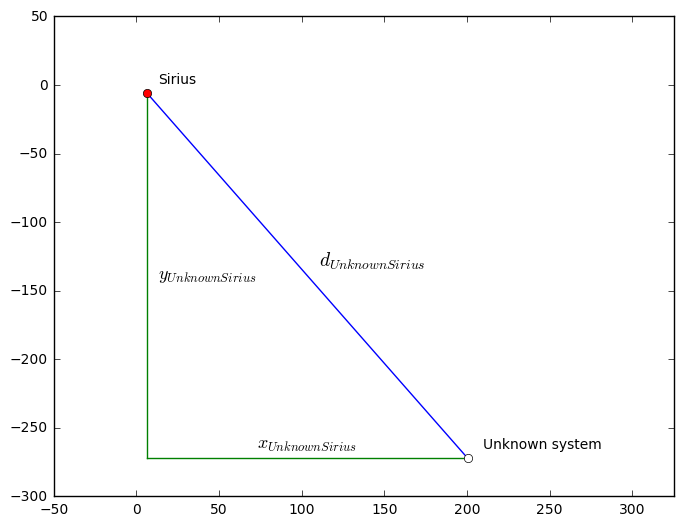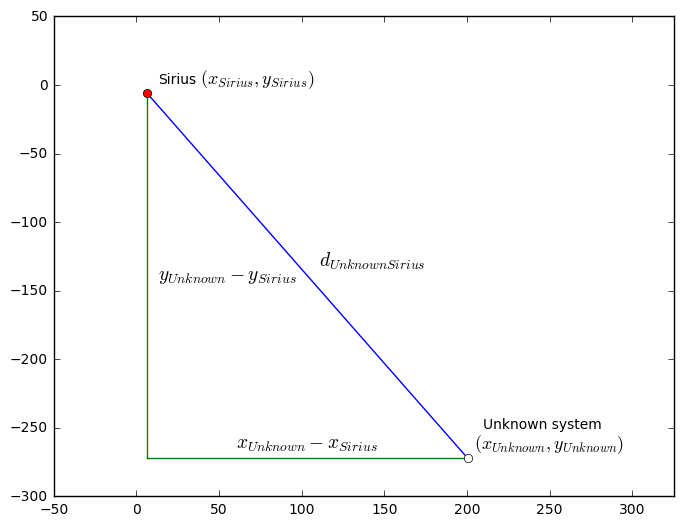# Solving the Unknown Probe reference system

## The math explained

August 21st 2016

### Foreword

When I published the trilateration results there were several people showing interest in how I came up with the results on both the Frontier forums and later Reddit. I posted the full math behind the trilateration but the complex equations made it intimidating for most people which was evident especially in the Reddit comments.

Despite the scary looking equations I feel that the basic math involved is rather simple and the necessary concepts are taught in high school in most countries. This article is my attempt at showing how simple concepts can be combined to calculate results that seem way complex.

While I know this probably won't be the most popular post on a gaming-related subreddit, as long as this helps even one person to understand math better or inspires them to approach it in a different way, I feel like spending the time to write this was worth it.

Also despite the common belief, I don't have a degree in mathematics. Nor did I receive my high school education in English. I'm confident most of the terms and concepts below are correct but I won't claim it's error proof. Corrections can be left on the Reddit post.

## Premise in 2D

When the Unknown Probe is deployed near a planet, it transmits a distance between the planet and an unknown system (among other things) using an unknown unit of length. For convenience let's name this unit a Bob. While we know that the Probe uses Merope (5C) as a reference planet and 1 Bob is the distance between the unknown system and Merope, we have no way of telling how many light years one Bob is without finding the unknown system. We can still use it to compare planets though: A planet with a reported distance of 0.8 Bobs is twice as far from the target system compared to a planet with a reported distance of 0.4 Bobs.

The measurement from a single planet doesn't really tell us anything - but once we gather measurements from different planets we start getting some kind of a picture of the possible location of the reference system. For the calculations here we have measured the distance from three different systems: Sirius, Merope and Betelgeuse. These systems are shown in figure 1 below.

Note: The distance values presented in the figure below are not the real values from the probe. The values the probe transmits are specific to Elite's 3D-galaxy and cannot be used for a 2D-example. The values below have been calculated based on the locations of the known systems and the (now known) unknown system location in 2D.

Those who are interested in the original 3D-values can check the Python solution at the bottom of the article.Figure 1: Unknown Probe measurements in various systems.

## Equations

The thing about mathematics is that you can always write down some equations. Let's start with the obvious ones: Distance between the unknown system and the scanned systems. Since we don't really have any real numbers to do the calculations with, we need to first introduce some letters:

• Let's use $d$ for distance (in light years).
• $d_\text"UnknownSirius"$ is the distance from the unknown system to Sirius.
• Let's use $x$ and $y$ for the coordinates (in light years).
• $x_\text"UnknownSirius"$ is the distance on x-axis between the unknown system and Sirius.
• $y_\text"UnknownSirius"$ is the distance on y-axis between the unknown system and Sirius.

These symbols are shown in figure 2.Figure 2: Distance symbols.

Now given the Pythagorean theorem, we can write our first equations using the above notation. The equations are listed below.

 $d_\text"UnknownSirius"^2$ $= x_\text"UnknownSirius"^2 + y_\text"UnknownSirius"^2$ $d_\text"UnknownMerope"^2$ $= x_\text"UnknownMerope"^2 + y_\text"UnknownMerope"^2$ $d_\text"UnknownBetelgeuse"^2$ $= x_\text"UnknownBetelgeuse"^2 + y_\text"UnknownBetelgeuse"^2$
Equation 1: Distances with Pythagorean theorem.

That's a start! These groups of equations are called systems of equations in mathematics. The basic systems taught in high school are system of linear equations - what we have here is a system of polynomial equations (thanks to the exponents). Fortunately this doesn't change things too much.

### Eliminating coordinates

A general rule of thumb with systems of equations is that they are solvable when the amount of equations corresponds to the amount of unknown variables. This gives us our first obstacle. Looking at the equations above, each of them contains three unknown values: $d\text"..."$, $x\text"..."$ and $y\text"..."$. Doing more measurements and adding more equations for different planets just introduces more variables. So before we can solve the group we need to find a way to eliminate some of the variables.

Let's start with the coordinates. From our premise we know there is only one unknown coordinate: The location of the unknown system. The locations of the three known planetery systems are.. well.. known. So let's introduce these into our equations. First some more letters:

• $x_\text"Sirius"$ is the x-coordinate of Sirius.
• $y_\text"Sirius"$ is the y-coordinate of Sirius.

Now we can redraw figure 2 using these new variables. This is shown in figure 3.Figure 3: Distances with absolute coordinates.

Comparing the two figures (2 and 3), we can spot the following relationship:

$x_\text"UnknownSirius" = x_\text"Unknown" - x_\text"Sirius"$
$y_\text"UnknownSirius" = y_\text"Unknown" - y_\text"Sirius"$

We can apply this to the above equations by replacing all the $x\text"..."$ and $y\text"..."$ variables. This gives us the following equations:

 $d_\text"UnknownSirius"^2$ $= (x_\text"Unknown" - x_\text"Sirius")^2 + (y_\text"Unknown" - y_\text"Sirius")^2$ $d_\text"UnknownMerope"^2$ $= (x_\text"Unknown" - x_\text"Merope")^2 + (y_\text"Unknown" - y_\text"Merope")^2$ $d_\text"UnknownBetelgeuse"^2$ $= (x_\text"Unknown" - x_\text"Betelgeuse")^2 + (y_\text"Unknown" - y_\text"Betelgeuse")^2$
Equation 2: Distances using absolute coordinates.

While this made the equation more complicated, the important difference is that it eliminated a large amount of unknown variables. Previously each equation had a different unknown $x$ and $y$ variable. Now all the equations share the same unknown $x_\text"Unknown"$ and $y_\text"Unknown"$ variables. All the other $x$ and $y$ variables are known and we don't need to solve them. We could substitute them with the numerical values (from figure 1), but we'll leave them like this for now just for clarity (I guess a personal preference).

### Introducing Bob-factor

We are still stuck with various $d\text"..."$ variables in the equation. The probe gave us distance values in the units we named Bob. We still don't know how many light years a single Bob is - but we can always come up with a letter for that amount as well:

• $r_\text"bob"$ is the amount of light years corresponding to one Bob.
• $b$ Bobs = $br_\text"bob"$ light years.
• $b_\text"UnknownSirius"$ is the distance between Sirius and the unknown system in Bobs.
• $b_\text"UnknownSirius"r_\text"bob"$ is the distance between Sirius and the unknown system in light years.

This allows us to write more equations.

 $d_\text"UnknownSirius"$ : Distance between Sirius and the unknown system in light years. $b_\text"UnknownSirius"$ : Distance between Sirius and the unknown system in Bobs. $d_\text"UnknownSirius"$ $= b_\text"UnknownSirius"r_\text"bob"$
Equation 3: Relationship between Bobs and light years.

Now since we know (or can decode) all the $b\text"..."$ values for the planets, we can finally get rid of the various unknown $d\text"..."$ values in our system of equations. Replacing the $d\text"..."$ values with $b\text"..."r_\text"bob"$ gives us the following equations:

 $(b_\text"UnknownSirius"r_\text"bob")^2$ $= (x_\text"Unknown" - x_\text"Sirius")^2 + (y_\text"Unknown" - y_\text"Sirius")^2$ $(b_\text"UnknownMerope"r_\text"bob")^2$ $= (x_\text"Unknown" - x_\text"Merope")^2 + (y_\text"Unknown" - y_\text"Merope")^2$ $(b_\text"UnknownBetelgeuse"r_\text"bob")^2$ $= (x_\text"Unknown" - x_\text"Betelgeuse")^2 + (y_\text"Unknown" - y_\text"Betelgeuse")^2$
Equation 4: Distance equations based on Bob measurements.

### Final numbers

Finally we have 3 equations with 3 unknown variables: $r_\text"bob"$, $x_\text"Unknown"$ and $y_\text"Unknown"$. This should make the equations solvable!

The only missing steps are inserting the known values to the equations and finding something to solve it with.

 Coordinates from EDSM $(x_\text"Sirius", y_\text"Sirius")$ $= (6.25, -5.75)$ $(x_\text"Merope", y_\text"Merope")$ $= (-78.1, -340.5)$ $(x_\text"Betelgeuse", y_\text"Betelgeuse")$ $= (169.4, -462.6)$ Distance measurements decoded from the probe. $b_\text"UnknownSirius"$ $= 1.149$ $b_\text"UnknownMerope"$ $= 1.000$ $b_\text"UnknownBetelgeuse"$ $= 0.673$
 $(1.149 r_\text"bob")^2$ $= (x_\text"Unknown" - 6.25)^2 + (y_\text"Unknown" - -5.75)^2$ $(1.000 r_\text"bob")^2$ $= (x_\text"Unknown" - -78.1)^2 + (y_\text"Unknown" - -340.5)^2$ $(0.673 r_\text"bob")^2$ $= (x_\text"Unknown" - 169.4)^2 + (y_\text"Unknown" - -462.6)^2$
Equation 5: Final equations with numerical values.

And finally typing that last part into Wolfram Alpha will give us the result.

... well, actually two candidates. Polynomial equations (Having exponents like $^2$ or $^3$) often yield multiple values. To figure out which one of these candidates is the real system, we need one more reference value. By replacing the equation values of Belegeuse with those of Sol ($b=1.156$, $(x,y) = (0,0)$) we can write the equations for Sirius-Merope-Sol combination to Wolfram Alpha. This gives us another result.

Only the candidate around $(x,y) = (688, -698)$ was common for these calculations.

### Differences in 3D

Unlike the above calculations, Elite Dangerous (and our galaxy in general) is a 3D system. This makes surprisingly little difference though. The two big differences are the ways coordinates are represented and distances calculated:

 System coordinates. $(x, y)$ $→$ $(x, y, z)$ Pythagorean Theorem $d^2 = x^2 + y^2$ $→$ $d^2 = x^2 + y^2 + z^2$

Like above, everything else follows from these basics. I won't go through the motions to derive the final equations in depth like I did above - the only difference in there being the additional z-term deriving straight from the change to the Pythagorean theorem:

 Final equations $(b\text"..."r_\text"bob")^2 = (x_\text"Unknown" - x\text"...")^2 + (y_\text"Unknown" - y\text"...")^2$ $→$ $(b\text"..."r_\text"bob")^2 = (x_\text"Unknown" - x\text"...")^2 + (y_\text"Unknown" - y\text"...")^2 + (z_\text"Unknown" - z\text"...")^2$

The additional z-coordinate adds one more unknown variable to the final equations: $z_\text"Unknown"$. The only practical difference this causes is that we now need one additional reference point:

4 unknown values - 4 equations - 4 reference systems.

### Tooling

There's one nasty caveat though: As the equations grow more complex, simple tools like Wolfram Alpha become a bit limited. For example while solving this originally I encountered a problem where the equation was too long (more than 200 characters) and it couldn't be entered to Wolfram Alpha. This doesn't mean these cannot be solved though - there are various free alternatives for getting the final numbers out of the math without having to pick up a pen and paper:

... and this is where it turns nasty. While I still feel the math above can be understood with a background of high school maths, the tools listed above might need a university degree to operate. :)

## SciPy script for solving the equations in 3D

Out of the options listed above, I chose SciPy to calculate the final values for the equations. The script I used (or a cleaned up version of it anyway) is included below:

from scipy.optimize import fsolve

# Coordinates from EDSM
xMerope, zMerope, yMerope = ( -78.59375, -149.625, -340.53125 )
xSynuefe, zSynuefe, ySynuefe = ( 74.53125, -149.375, -339.96875 )
xCol285, zCol285, yCol285 = ( -78.65625, -150.59375, -49.25 )
xWredguia, zWredguia, yWredguia = ( -78, 175.8125, -341.125 )

# Distances in Bobs
bMerope = 1
bSynuefe = 0.85
bCol285 = 1.177
bWredguia = 1.149

# Define a function that evaluates the equations
def equations( guess ):
r, x, z, y = guess

return (
( x - xMerope )**2   + ( y - yMerope )**2   + ( z - zMerope )**2   - ( bMerope * r )**2,
( x - xSynuefe )**2  + ( y - ySynuefe )**2  + ( z - zSynuefe )**2  - ( bSynuefe * r )**2,
( x - xCol285 )**2   + ( y - yCol285 )**2   + ( z - zCol285 )**2   - ( bCol285 * r )**2,
( x - xWredguia )**2 + ( y - yWredguia )**2 + ( z - zWredguia )**2 - ( bWredguia * r )**2,
)

# Make SciPy solve the system using an initial guess.
# The initial guess affects which of the "candidates" SciPy finds.
initial_guess = ( 0, 5000, -5000, -5000 )
result = fsolve( equations, initial_guess )

# Display the results.
print( "(r, x, z, y) =", result )

Output:
    (r, x, z, y) = [
871.01391722
687.07099255
-362.1818179
-697.24170006
]

### Afterword

The analysis above described how simple concepts of Pythagorean theorem and equation systems can be applied together to calculate locations of unknown star systems. The article started with the theory in 2D and then generalized this to 3D by introducing the 3D-version of the Pythagorean theorem.

My hope is that at at least few people reading this will finally realize that learning hundreds of different formulas and equations is not important in mathematics. The important thing is to figure out how to apply the few you know to the problems at hand.

- Mikko Rantanen / CMDR Wace

Any questions and comments can be left on the Reddit post or directly to /u/Wace.